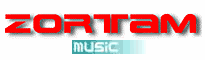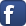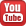Artist: A B C D E F G H I J K L M N O P Q R S T U V W X Y Z 0-9Songs    | DOWNLOAD NOW!    | Albums    | Album ArtsSong: Gypsy Album: Saint Dominic's Preview Genres: Rock Year: 1972 Length: 257 sec

Lyrics:

You can make out pretty good
And you know just where you are
When you wanna roam
Pull into a wooded glen

La da di di di di di
La da di di di di di
La da di di di di di daaa
La da di di di di di
La da di di di di di
La da di di di di di daaa
Gypsy!

Laying underneath the stars
Can be so much fun
Especially when you're feeling good
When you're with the one you love

Sway to sounds of two guitars
Around the campfire bright
Then mellow out like violins
In the morning light

La da di di di di di
La da di di di di di
La da di di di di di daaa
La da di id di di di
La da di di di di di daaa
Gypsy!

No matter where you wander
And no matter where you roam
Any place you hang your hat
You know that that is home, check it out first

Sway to sounds of two guitars
Around the campfire bright
Then mellow out like old violins
In the morning light

La da di di di di di
La da di di di di di
La da di di di di di daaa, hep
La da di di di di di
La da di di di di di
La da di di di di di daaa
Gypsy!

La da di di di di di
La da di di di di di
La da di di di di di daaaa, hep
La da di di di di di
La da di di di di di
La da di di di di di daaa
GypsyCopyright © 2019 Zortam.com. All Rights Reserved.